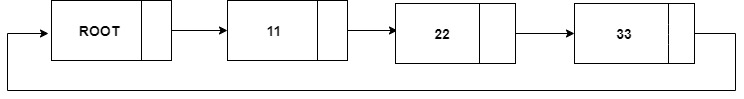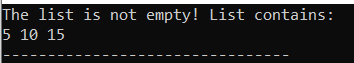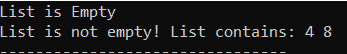×

Search anything:

# Check if Linked List is Empty

#### Algorithms linked list Data StructuresLinked list is a linear data structure used to store elements of similar data type.
Each node in a list consists of at least two parts:

1. data value
2. pointer to the next node

Linked list can be formed in two ways:

• with a root element
• without a root

Checking if the given Linked List is empty depends on the ways Linked List has been formed. We have covered both approaches.

# With a root

The root acts as an element which is always present even if the list is empty.
The other use of having a root in list is that we can link the last element back to the root forming a cycle. According to this concept, next of any node is never NULL.Pseudocode:

``````bool isEmpty(node *root){
if (root->next == root) return true;
else return false;
}
``````

The implementation is given below:

``````#include<bits/stdc++.h>
using namespace std;

class Node{

public:
int data;
Node *next;

Node(){
data=0;
next=NULL;
}
};

Node *root;
public:

root=NULL;
}

Node* getRoot(){
return root;
}

Node *temp = new Node();
temp->data = n;
temp->next = NULL;
if(root == NULL){
root=temp;
root->next=root;
}
else{
Node *last=root;
while(last->next!=root){
last=last->next;
}
temp->next=root;
last->next=temp;

}
}

void printList(){
Node *temp=root;
if(temp!=NULL){
do{
cout<<temp->data<<" ";
temp = temp->next;
} while(temp!=root);
}

}

bool isEmpty(){
if(root->next==root && root->data==0) return true;
return false;
}

};

int main(){

if(l1.isEmpty()) cout<<"The list is empty!\n";
else {
cout<<"The list is not empty! List contains:\n";
l1.printList();
}

return 0;
}
``````

Output:# without root

Without a root, the list pointer is NULL when the list is empty.
Pseudocode:

``````bool isEmpty(node *list){
if (list == NULL) return true;
else return false;
}
``````

Implementation of Linked List without root is given below:

``````#include<bits/stdc++.h>
using namespace std;

class Node
{
public:
int data;
Node *next;
} ;

Node* new_node = new Node();
new_node->data = new_data;
}

bool isEmpty( Node** list){
if((*list)==NULL) return true;
return false;
}

void printList(Node *node)
{
while (node != NULL)
{
cout<<node->data<<" ";
node = node->next;
}
}

int main(){

Node *list=NULL;
if(isEmpty(&list)) cout<<"List is Empty"<<endl;
else{
cout<<"List is not empty! List contains:"<<" ";
printList(list);
}
// Inserting some elements in list
push(&list,8);
push(&list,4);

if(isEmpty(&list)) cout<<"List is Empty"<<endl;
else{
cout<<"List is not empty! List contains:"<<" ";
printList(list);
}
return 0;
}
``````

Output:Complexity:
The complexity to check whether the list is empty or not in both the approaches is same i.e O(1)